Prashant Sahu — Published On January 27, 2023 and Last Modified On January 27th, 2023

## Introduction

Ordinary Least squares is an optimization technique. OLS is the same technique that is used by the scikit-learn LinearRegression class and the numpy.polyfit() function behind the scenes. Before we proceed into the details of the OLS technique, it would be worthwhile going through the article I have written on the role of Optimization techniques in machine learning & deep learning. In the same article, I have briefly explained the reason and context for the existence of the OLS technique (Section 6). This article is very much in continuation of the same article, and readers are expected to be familiar with the same.Source: Pixbay

Learning Objectives:

1. Learn what OLS is and understand its mathematical equation
2. Get an overview of OLS in scaler form and its drawbacks
3. Understand OLS using a real-time example

1. What are optimization problems?
2. Why do we need OLS?
3. Understanding the Mathematics Behind the OLS Algorithm
4. OLS Solution in Scaler form
5. OLS in Action using an actual example
6. Problems with the Scaler form of OLS Solution
7. Conclusion

## What are Optimization Problems?

Optimization problems are mathematical problems that involve finding the best solution from a set of possible solutions. These problems are typically formulated as maximization or minimization problems, where the goal is to either maximize or minimize a certain objective function. The objective function is a mathematical expression describing the quantity to be optimized, and a set of constraints defines the set of possible solutions.

Optimization problems arise in various fields, including engineering, finance, economics, and operations research. They are used to model and solve problems such as resource allocation, scheduling, and portfolio optimization. Optimization is a crucial component of many machine learning algorithms. In machine learning, optimization is used to find the best set of parameters for a model that minimizes the difference between the model’s predictions and the true values. Optimization is an active area of research in machine learning, with new optimization algorithms being developed to improve the speed and accuracy of training machine learning models.

Some examples of where optimization is used in machine learning include:

• In supervised learning, optimization is used to find the parameters of a model that minimize the difference between the model’s predictions and the true values for a given training dataset. For example, linear regression and logistic regression use optimization to find the best values of the model’s coefficients. In addition, some models like decision trees, random forests, and gradient boosting models are built by iteratively adding new models to the ensemble and optimize the parameters of the new models that minimize the error on the training data.
• In unsupervised learning, optimization helps to find the best configuration of clusters or mapping of the data that best represents the underlying structure in the data. In clustering, optimization is used to find the best configuration of clusters in the data. For example, the K-Means algorithm uses an optimization technique called Lloyd’s algorithm, which iteratively reassigns data points to the nearest cluster centroid and updates the cluster centroids based on the newly assigned points. Similarly, other clustering algorithms such as hierarchical clustering, density-based clustering, and Gaussian mixture models also use optimization techniques to find the best clustering solution. In dimensionality reduction, optimization finds the best data mapping from a high- to a lower-dimensional space. For example, Principal Component Analysis (PCA) uses Singular Value Decomposition (SVD), an optimization technique, to find the best linear combination of the original variables that explains the most variance in the data. Additionally, other dimensionality reduction techniques like Linear Discriminant Analysis (LDA) and t-distributed Stochastic Neighbor Embedding (t-SNE) also use optimization techniques to find the best representation of the data in a lower-dimensional space.
• In deep learning, optimization is used to find the best set of parameters for neural networks, which is typically done using gradient-based optimization algorithms such as stochastic gradient descent (SGD) or Adam/Adagrad/RMSProp, etc.

## Why do we Need OLS?

The ordinary least squares (OLS) algorithm is a method for estimating the parameters of a linear regression model. The OLS algorithm aims to find the values of the linear regression model’s parameters (i.e., the coefficients) that minimize the sum of the squared residuals. The residuals are the differences between the observed values of the dependent variable and the predicted values of the dependent variable given the independent variables. It is important to note that the OLS algorithm assumes that the errors are normally distributed with zero mean and constant variance and that there is no multicollinearity (high correlation) among the independent variables. Other methods, such as Generalized Least Squares or Weighted Least Squares, should be used in cases where these assumptions are not met.

## Understanding the Mathematics behind the OLS Algorithm

To explain the OLS algorithm, let me take the simplest possible example. Consider the following 3 data points: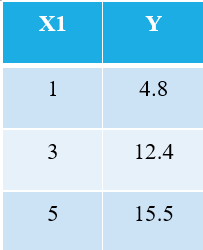Everyone familiar with machine learning will immediately recognize that we are referring to X1 as the independent variable (also called “Features” or “Attributes”), and the Y is the dependent variable (also referred to as the “Target” or “Outcome”). Hence, the overall task of any machine is to find the relationship between X1 & Y. This relationship is actually “learned” by the machine from the DATA. Hence, we call the term Machine Learning. We, humans, learn from our experiences. Similarly, the same experience is fed into the machine as data.

Now, let’s say we want to find the best-fit line through the above 3 data points. The following plot shows these 3 data points in blue circles. Also shown is the red line (with squares), which we claim as the “best-fit line” through these 3 data points. Also, I have shown a “poor-fitting” line (the yellow line) for comparison.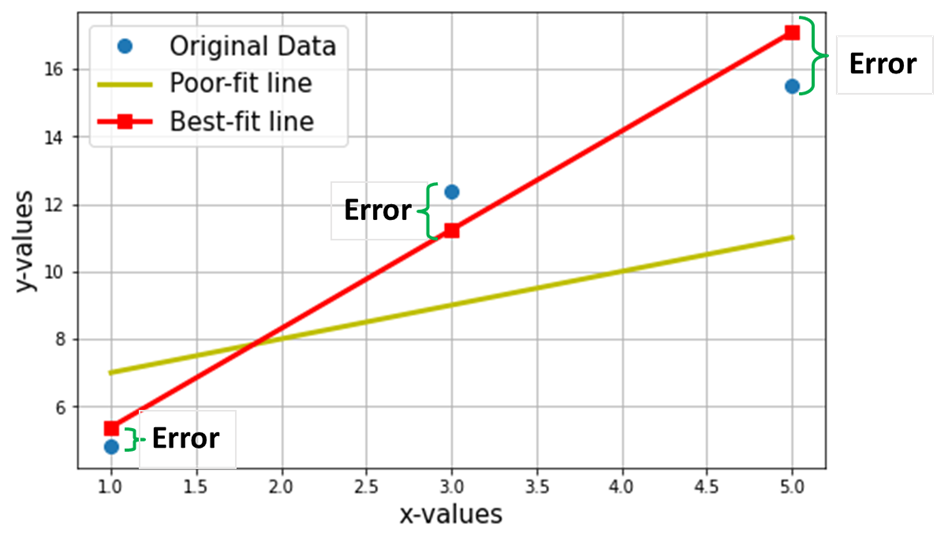The net objective is to find the Equation of the Best-Fitting Straight Line (through these 3 data points mentioned in the above table).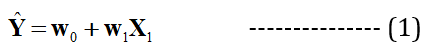It is the equation of the best-fit line (red line in the above plot), where w1 = slope of the line; w0 = intercept of the line.
In machine learning, this best fit is called the Linear Regression (LR) model, and w0 and w1 are also called model weights or model coefficients.

Red-squares in the above plot represent the predicted values from the Linear Regression model (Y^). Of course, the predicted values are NOT the same as the actual values of Y (blue circles). The vertical difference represents the error in the prediction given by (see the image below) for any ith data point.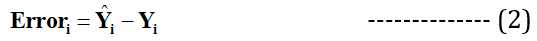Now I claim that this best fit-line will have the minimum error for prediction (among all possible infinite random “poor-fit” lines). This total error across all the data points is expressed as the Mean Squared Error  (MSE) Function, which will be the minimum for the best-fit line.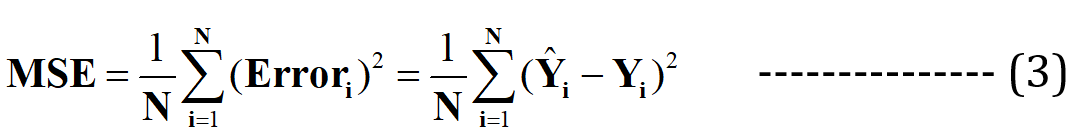N = Total no. of data points in the dataset (in the current case, it is 3)
Minimizing or maximizing any quantity is mathematically referred to as an Optimization Problem, and hence the solution (the point where the minimum/maximum exists) refers to the variables’ optimal values.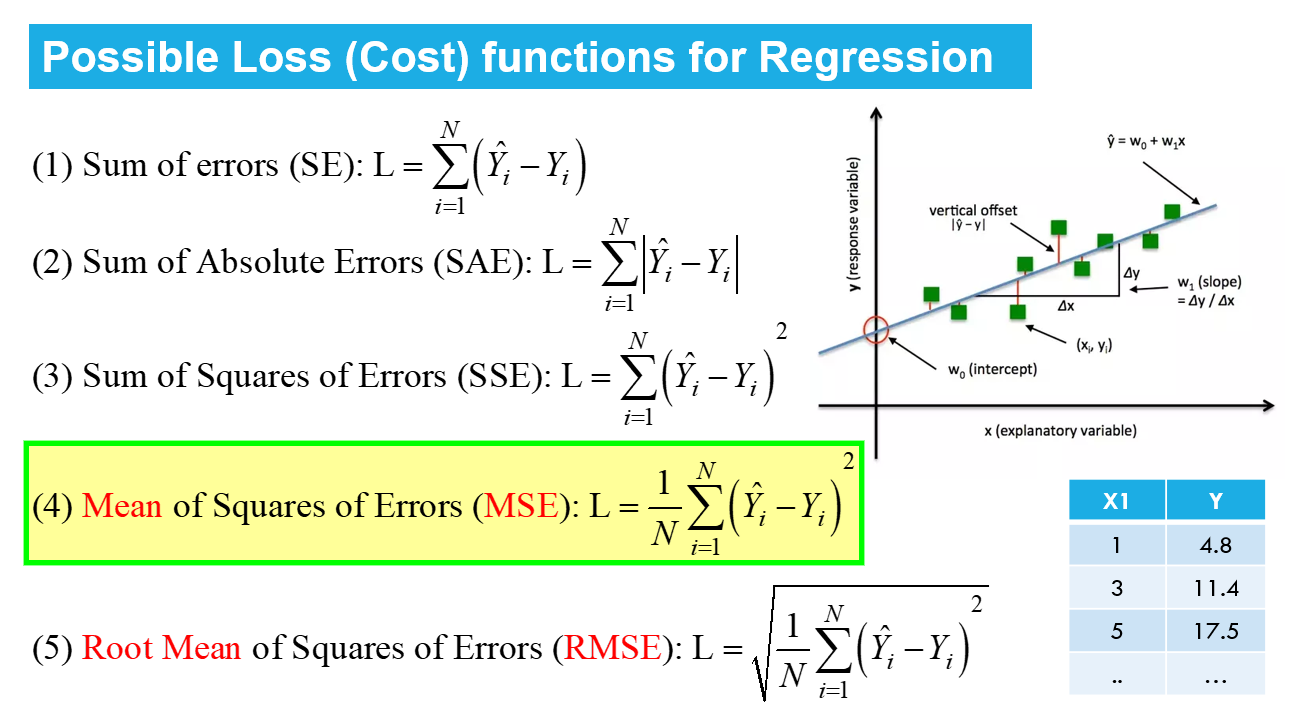Linear Regression is an example of unconstrained optimization, given by: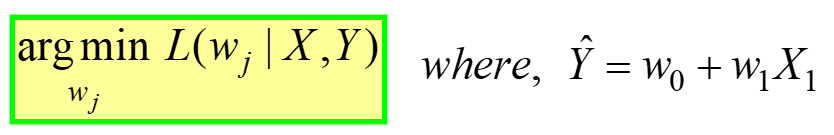———– (4)

This is read as “Find the optimal weights (wj) for which the MSE Loss function (given in eq. 3 above) has min value, for a GIVEN X, Y data(refer to very first table at the start of the article). L(wj) represents the MSE Loss, a function of the model weights, not X or Y. Remember, X & Y is your DATA and is supposed to be CONSTANT! The subscript “j” represents the jth model coefficient/weight.

Upon substituting for Y^ = w0 + w1Xin the eq. 3 above, the final MSE Loss Function (L) looks as: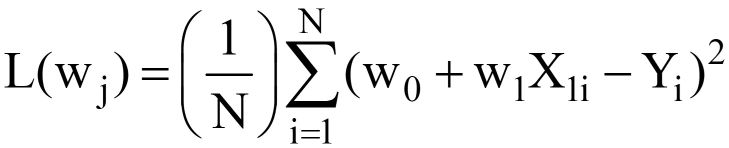———– (5)

Clearly, L is a function of model weights (w0 & w1), whose optimal values we have to find upon minimizing L. The optimal values are represented by (*) in the figure below.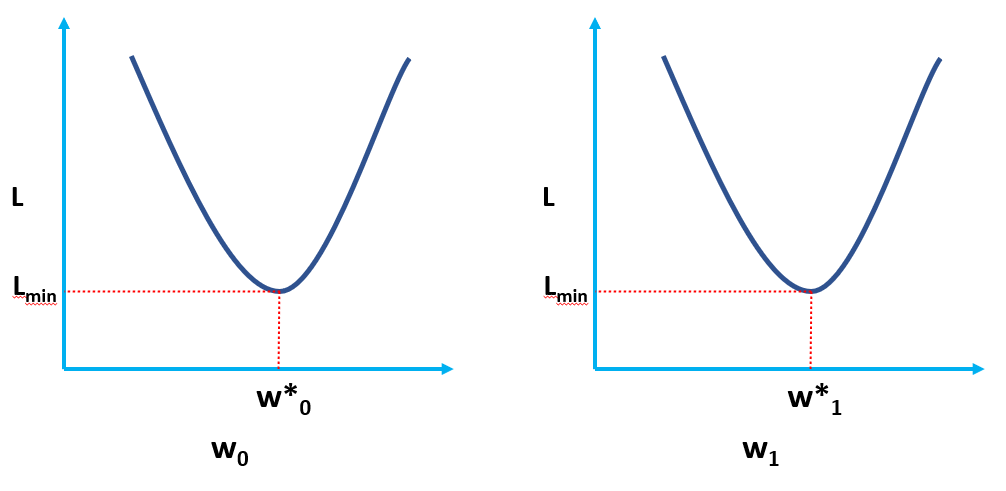## OLS Solution in Scaler Form

The eq. 5 given above represents the OLS Loss function in the scaler form (where we can see the summation of errors for each data point. The OLS algorithm is an analytical solution to the optimization problem presented in the eq. 4. This analytical solution consists of the following steps:

Step 1: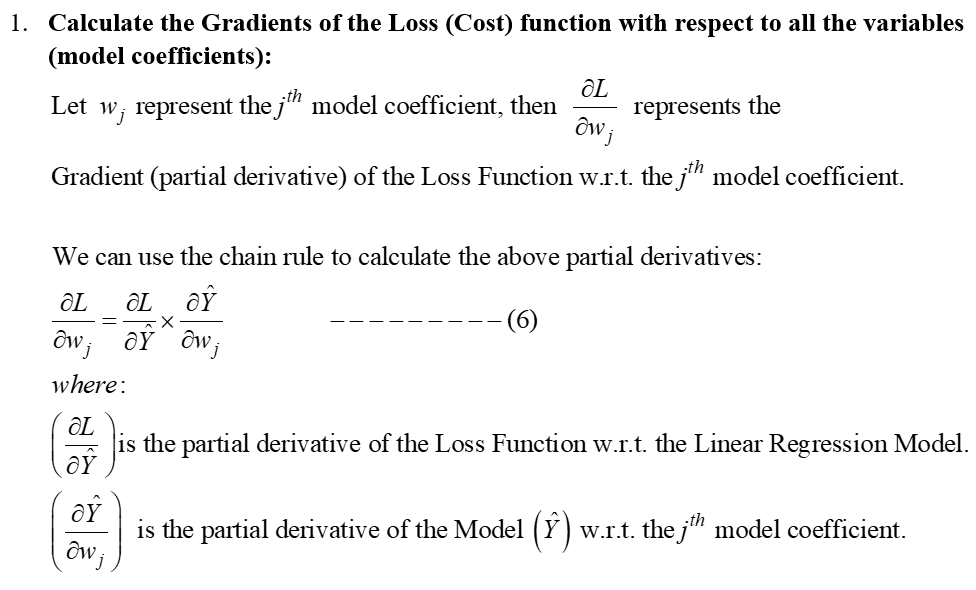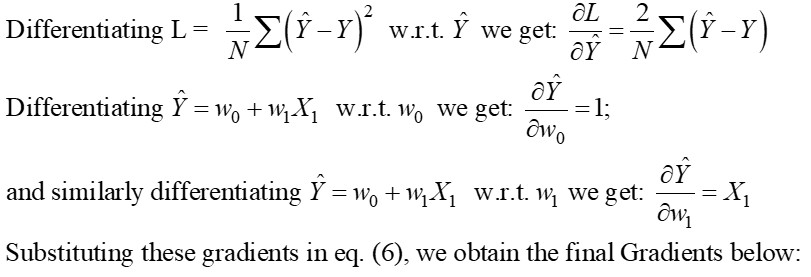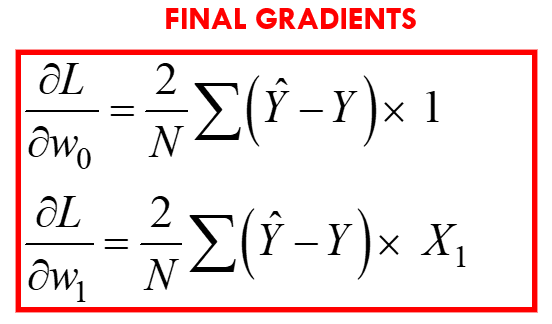Step 2: Equate these gradients to zero and solve for the optimal values of the model coefficients wj.

This basically means that the slope of the tangent (the geometrical interpretation of the gradients) to the Loss function at the optimal values (the point where L is minimum) will be zero, as shown in the figures above.From the above equations, we can shift the “2” from the LHS to the RHS; the RHS remains as 0 (as 0/2 is still 0).These expressions for w1* and w0* are the final OLS Analytical solution in the Scaler form.

Step 3: Compute the above means and substitute in the expression for w1* & w0*.

Let’s calculate these values for our dataset: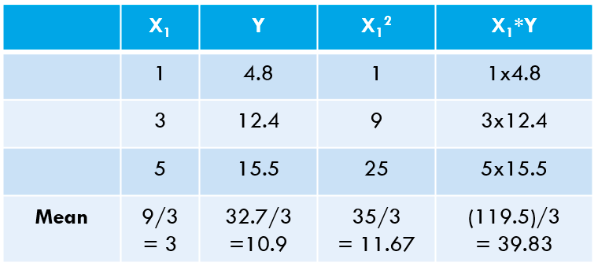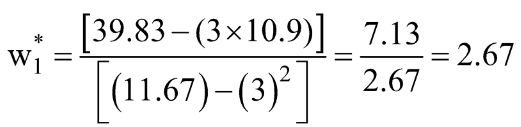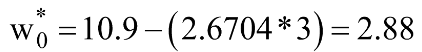Let us calculate the same using Python code:

```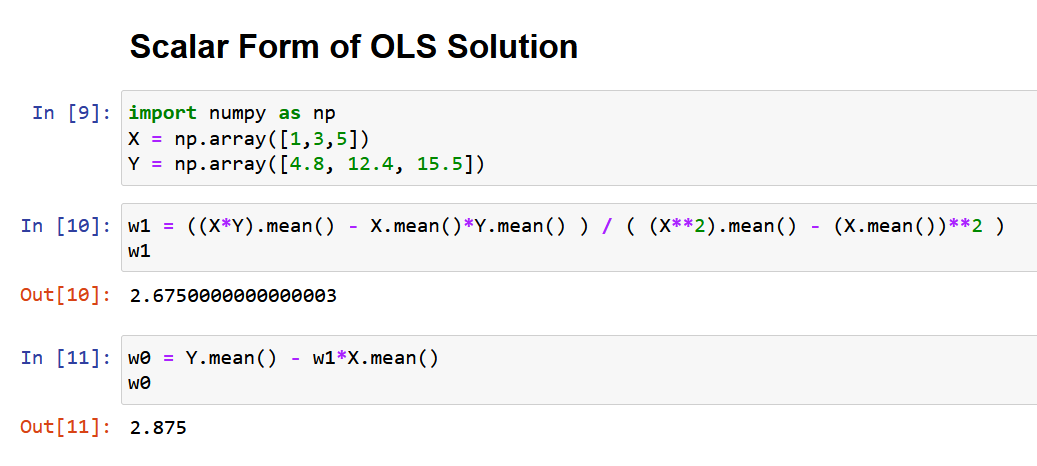[OUTPUT]: This is the Equation of the "Best-fit" Line:
2.675 x + 2.875
```

You can see our “hand-calculated” values match very closely the values of slope and intercept obtained using NumPy (the small difference is due to round-off errors in our hand-calculations). We can also verify that the same OLS is “running behind the scenes” of the LinearRegression class from the scikit-learn package, as demonstrated in the code below.

```# import the LinearRegression class from scikit-learn package
from sklearn.linear_model import LinearRegression

LR = LinearRegression() # create an instance of the LinearRegression class

# define your X and Y as NumPy Arrays (column vectors)
X = np.array([1,3,5]).reshape(-1,1)
Y = np.array([4.8,12.4,15.5]).reshape(-1,1)

LR.fit(X,Y) # calculate the model coefficients
LR.intercept_ # the bias or the intercept term (w0*)```
`[Output]: array([2.875])`
```LR.coef_ # the slope term (w1*)
[Output]: array([[2.675]])
```

## OLS in Action Using an Actual Example

Here I am using the Boston House Pricing dataset, one of the most commonly encountered datasets while learning Data Science. The objective is to make a Linear Regression Model to Predict the median value of the House prices based on 13 features/attributes mentioned below.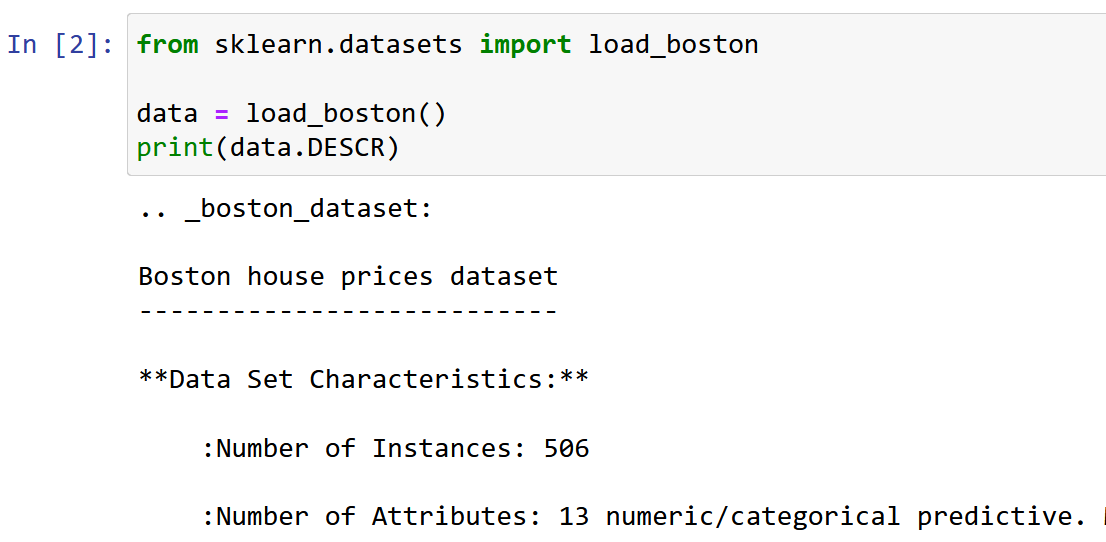Import and explore the dataset.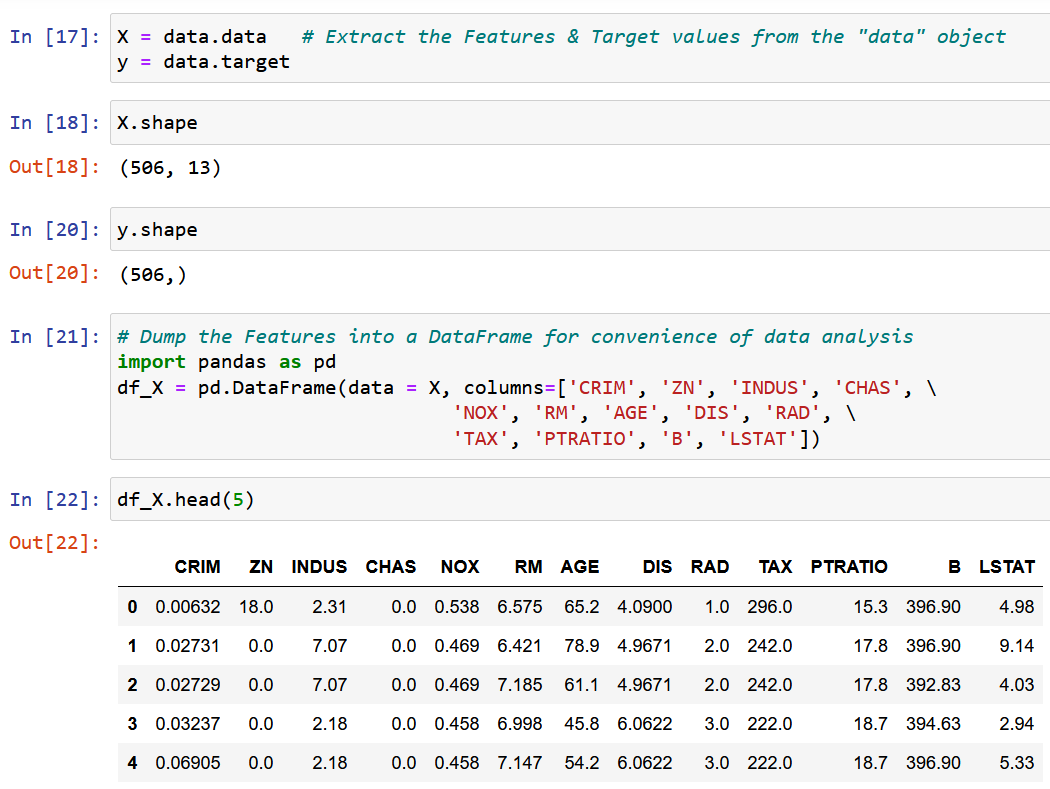We’ll extract a single feature RM, the average room size in the given locality, and fit it with the target variable y (the median value of the house price).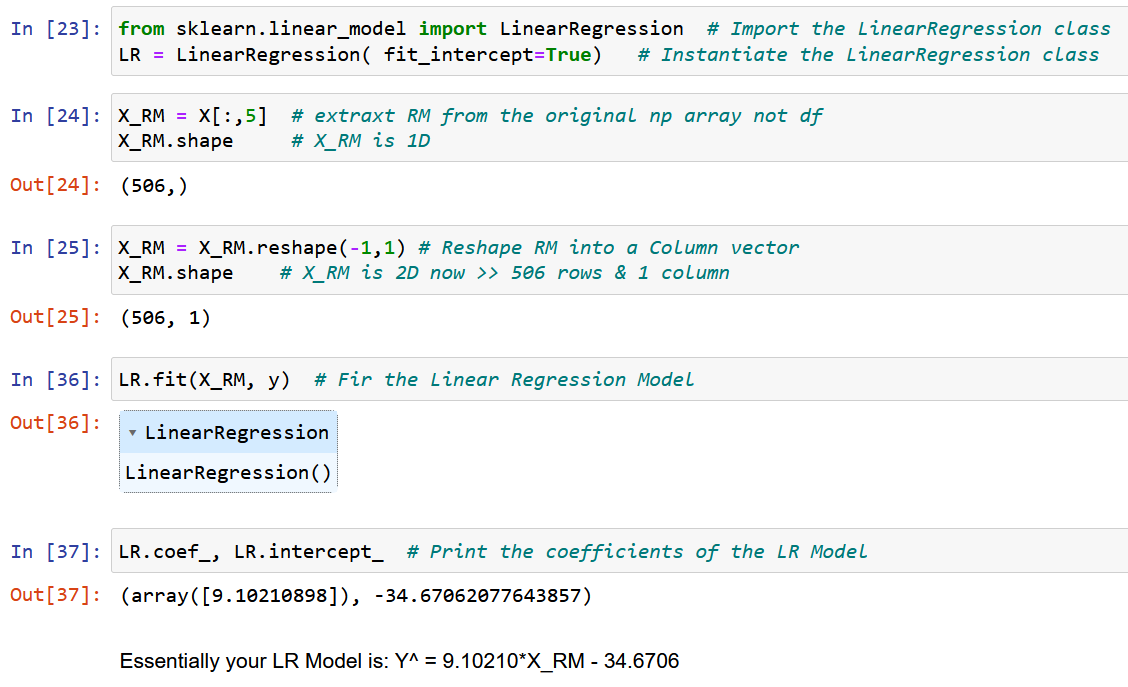Now, let’s use pure NumPy and calculate the model coefficients using the expressions derived for the optimal values of the model coefficients w0 & w1 above (end of Step 2).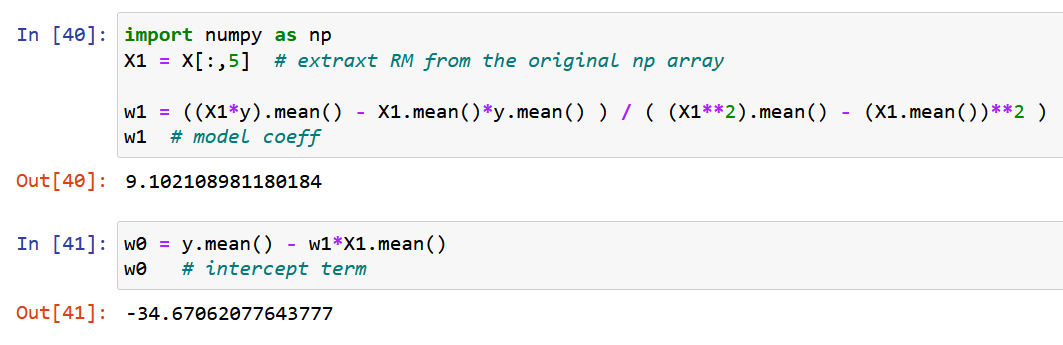Let us finally plot the original data along with the best-fit line, as given below.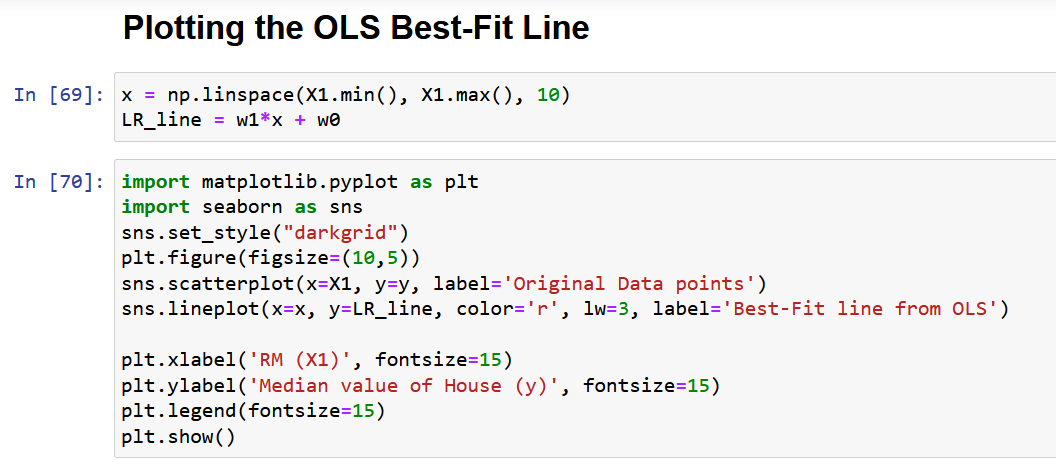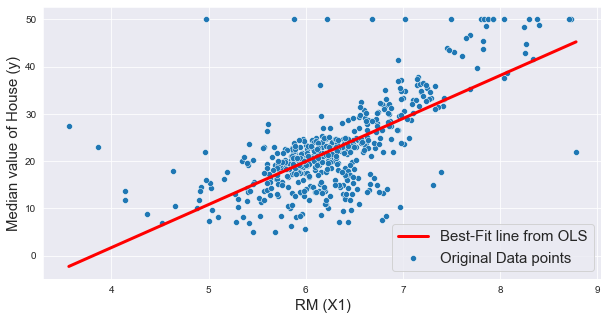## Problems with the Scaler Form of OLS Solution

Finally, let me discuss the main problem with the above approach, as described in section 4. As you can see from the abovementioned dataset, any real-life dataset will have multiple features. The main reason I have taken only one feature for demonstrating the OLS method in the above section was that as the number of features increase, the number of gradients would also increase, hence the number of equations to be solved simultaneously!

To be exact, for 13 features (Boston dataset above), we’ll have 13 model coefficients and one intercept term, which brings the total number of variables to be optimized to 14. Hence, we’ll obtain 14 gradients (the partial derivative of the loss function with respect to each of these 14 variables). Consequently, we need to solve 14 equations (after equating these 14 partial derivatives to zero, as described in step 2). You have already realized the complexity of the analytical solution with just 2 variables. Frankly, I have tried to give you the MOST elaborate explanation of OLS available on the internet, and yet it is not easy to assimilate the mathematics.

Hence, in simple words, the above analytical solution is NOT SCALABLE!

The solution to this problem is the “Vectorized Form of the OLS Solution,” which will be discussed in detail in a follow-up article (Part 2 of this article), with sections 7 & 8.

## Conclusion

In conclusion, the OLS method is a powerful tool for estimating the parameters of a linear regression model. It is based on the principle of minimizing the sum of squared differences between the predicted and actual values.

Some of the key takeaways from the article are as follows:

• The OLS solution can be represented in scaler form, making it easy to implement and interpret.
• The article discussed the concept of optimization problems and the need for OLS in regression analysis and provided a mathematical formulation and an example of OLS in action.
• The article also highlights some of the limitations of the scaler form of the OLS solution, such as scalability and the assumptions of linearity and constant variance. I hope you got to learn something new from this article.

Please drop me a comment if you feel any point/equation in this article needs explanation or if you want me to write about any other machine learning algorithm in such detail.